Custom SearchFigure 3-12. - Operational amplifier.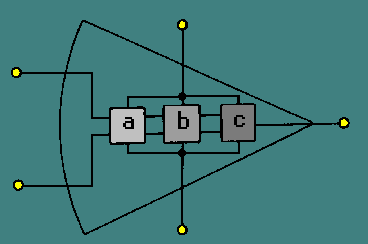CLOSED-LOOP OPERATION OF AN OPERATIONAL AMPLIFIER Operational amplifiers can have either a closed-loop operation or an open-loop operation. The operation (closed-loop or open-loop) is determined by whether or not feedback is used. Without feedback the operational amplifier has an open-loop operation. This open-loop operation is practical only when the operational amplifier is used as a comparator (a circuit which compares two input signals or compares an input signal to some fixed level of voltage). As an amplifier, the open-loop operation is not practical because the very high gain of the operational amplifier creates poor stability. (Noise and other unwanted signals are amplified so much in open-loop operation that the operational amplifier is usually not used in this way.) Therefore, most operational amplifiers are used with feedback (closed-loop operation). Operational amplifiers are used with degenerative (or negative) feedback which reduces the gain of the operational amplifier but greatly increases the stability of the circuit. In the closed-loop configuration, the output signal is applied back to one of the input terminals. This feedback is always degenerative (negative). In other words, the feedback signal always opposes the effects of the original input signal. One result of degenerative feedback is that the inverting and noninverting inputs to the operational amplifier will be kept at the same potential. Closed-loop circuits can be of the inverting configuration or noninverting configuration. Since the inverting configuration is used more often than the noninverting configuration, the inverting configuration will be shown first. Inverting Configuration Figure 3-13 shows an operational amplifier in a closed-loop, inverting configuration. Resistor R2 is used to feed part of the output signal back to the input of the operational amplifier. Figure 3-13. - Inverting configuration.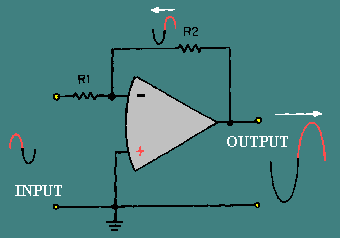At this point it is important to keep in mind the difference between the entire circuit (or operational circuit) and the operational amplifier. The operational amplifier is represented by the triangle-like symbol while the operational circuit includes the resistors and any other components as well as the operational amplifier. In other words, the input to the circuit is shown in figure 3-13, but the signal at the inverting input of the operational amplifier is determined by the feedback signal as well as by the circuit input signal. As you can see in figure 3-13, the output signal is 180 degrees out of phase with the input signal. The feedback signal is a portion of the output signal and, therefore, also 180 degrees out of phase with the input signal. Whenever the input signal goes positive, the output signal and the feedback signal go negative. The result of this is that the inverting input to the operational amplifier is always very close to 0 volts with this configuration. In fact, with the noninverting input grounded, the voltage at the inverting input to the operational amplifier is so small compared to other voltages in the circuit that it is considered to be VIRTUAL GROUND. (Remember, in a closed-loop operation the inverting and noninverting inputs are at the same potential.) Virtual ground is a point in a circuit which is at ground potential (0 volts) but is NOT connected to ground. Figure 3-14, (view A) (view B) and (view C), shows an example of several circuits with points at virtual ground. Figure 3-14A. - Virtual ground circuits.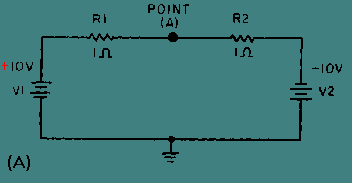Figure 3-14B. - Virtual ground circuits.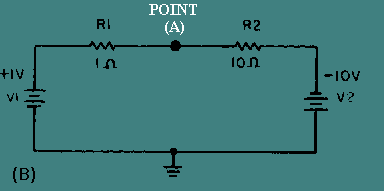Figure 3-14C. - Virtual ground circuits.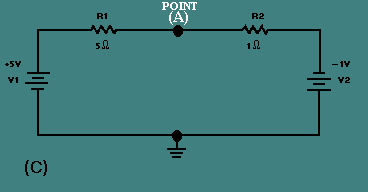In view (A), V1 (the left-hand battery) supplies +10 volts to the circuit while V2 (the right-hand battery) supplies -10 volts to the circuit. The total difference in potential in the circuit is 20 volts. The total resistance of the circuit can be calculated: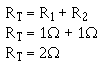Now that the total resistance is known, the circuit current can be calculated: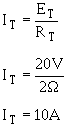The voltage drop across R1 can be computed: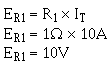The voltage at point A would be equal to the voltage of V1 minus the voltage drop of R1.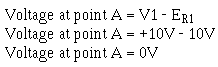To check this result, compute the voltage drop across R2 and subtract this from the voltage at point A. The result should be the voltage of V2.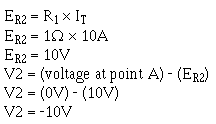It is not necessary that the voltage supplies be equal to create a point of virtual ground. In view (B) V1 supplies +1 volt to the circuit while V2 supplies -10 volts. The total difference in potential is 11 volts. The total resistance of this circuit (R1 + R2) is 11 ohms. The total current (IT) is 1 ampere. The voltage drop across R1 (E R1 = R1 X IT) is 1 volt. The voltage drop across R2 (ER2 = R2 X I T) is 10 volts. The voltage at point A can be computed: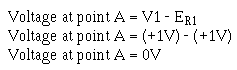So point A is at virtual ground in this circuit also. To check the results, compute the voltage at V2.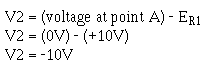Integrated Publishing, Inc. - A (SDVOSB) Service Disabled Veteran Owned Small Business